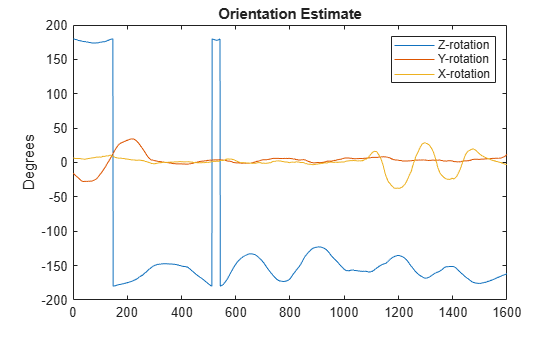# complementaryFilter

Orientation estimation from a complementary filter

## Description

The `complementaryFilter` System object™ fuses accelerometer, gyroscope, and magnetometer sensor data to estimate device orientation and angular velocity.

To estimate orientation using this object:

1. Create the `complementaryFilter` object and set its properties.

2. Call the object with arguments, as if it were a function.

## Creation

### Syntax

``FUSE = complementaryFilter``
``FUSE = complementaryFilter('ReferenceFrame',RF)``
``FUSE = complementaryFilter(___,Name,Value)``

### Description

````FUSE = complementaryFilter` returns a `complementaryFilter` System object, `FUSE`, for sensor fusion of accelerometer, gyroscope, and magnetometer data to estimate device orientation and angular velocity. ```
````FUSE = complementaryFilter('ReferenceFrame',RF)` returns a `complementaryFilter` System object that fuses accelerometer, gyroscope, and magnetometer data to estimate device orientation relative to the reference frame `RF`. Specify `RF` as `'NED'` (North-East-Down) or `'ENU'` (East-North-Up). The default value is `'NED'`.```

example

````FUSE = complementaryFilter(___,Name,Value)` sets each property `Name` to the specified `Value`. Unspecified properties have default values.```

## Properties

expand all

Unless otherwise indicated, properties are nontunable, which means you cannot change their values after calling the object. Objects lock when you call them, and the `release` function unlocks them.

If a property is tunable, you can change its value at any time.

Input sample rate of the sensor data in Hz, specified as a positive scalar.

Tunable: No

Data Types: `single` | `double`

Accelerometer gain, specified as a real scalar in the range of [0, 1]. The gain determines how much the accelerometer measurement is trusted over the gyroscope measurement for orientation estimation. This property is tunable.

Data Types: `single` | `double`

Magnetometer gain, specified as a real scalar in the range of [0, 1]. The gain determines how much the magnetometer measurement is trusted over the gyroscope measurement for orientation estimation. This property is tunable.

Data Types: `single` | `double`

Enable magnetometer input, specified as `true` or `false`.

Data Types: `logical`

Output orientation format, specified as `'quaternion'` or `'Rotation matrix'`. The size of the output depends on the output orientation format:

• `'quaternion'` –– Output is an N-by-1 `quaternion`.

• `'Rotation matrix'` –– Output is a 3-by-3-by-N rotation matrix.

N is the number of samples.

Data Types: `char` | `string`

## Usage

### Syntax

``[orientation,angularVelocity] = FUSE(accelReadings,gyroReadings,magReadings)``
``[orientation,angularVelocity] = FUSE(accelReadings,gyroReadings)``

### Description

example

````[orientation,angularVelocity] = FUSE(accelReadings,gyroReadings,magReadings)` fuses accelerometer, gyroscope, and magnetometer data to compute orientation and angular velocity. To use this syntax, set the `HasMagnetometer` property as `true`.```
````[orientation,angularVelocity] = FUSE(accelReadings,gyroReadings)` fuses accelerometer and gyroscope data to compute orientation and angular velocity. To use this syntax, set the `HasMagnetometer` property as `false`.```

### Input Arguments

expand all

Accelerometer readings in the sensor body coordinate system in m/s2, specified as an N-by-3 matrix. N is the number of samples, and the three columns of `accelReadings` represent the [x y z] measurements. Accelerometer readings are assumed to correspond to the sample rate specified by the `SampleRate` property. In the filter, the gravity constant g is assumed to be `9.81` m/s2.

Data Types: `single` | `double`

Gyroscope readings in the sensor body coordinate system in rad/s, specified as an N-by-3 matrix. N is the number of samples, and the three columns of `gyroReadings` represent the [x y z] measurements. Gyroscope readings are assumed to correspond to the sample rate specified by the `SampleRate` property.

Data Types: `single` | `double`

Magnetometer readings in the sensor body coordinate system in µT, specified as an N-by-3 matrix. N is the number of samples, and the three columns of `magReadings` represent the [x y z] measurements. Magnetometer readings are assumed to correspond to the sample rate specified by the `SampleRate` property.

Data Types: `single` | `double`

### Output Arguments

expand all

Orientation that rotates quantities from the local navigation coordinate system to the body coordinate system, returned as quaternions or an array. The size and type of `orientation` depends on whether the `OrienationFormat` property is set to `'quaternion'` or `'Rotation matrix'`:

• `'quaternion'` –– the output is an N-by-1 vector of quaternions, where N is the number of samples.

• `'Rotation matrix'` –– the output is a 3-by-3-by-N array of rotation matrices, where N is the number of samples.

Data Types: `quaternion` | `single` | `double`

Angular velocity expressed in the sensor body coordinate system in rad/s, returned as an N-by-3 array, where N is the number of samples.

Data Types: `single` | `double`

## Object Functions

To use an object function, specify the System object as the first input argument. For example, to release system resources of a System object named `obj`, use this syntax:

`release(obj)`

expand all

 `step` Run System object algorithm `release` Release resources and allow changes to System object property values and input characteristics `reset` Reset internal states of System object `clone` Create duplicate System object `isLocked` Determine if System object is in use

## Examples

collapse all

Load the `rpy_9axis` file, which contains recorded accelerometer, gyroscope, and magnetometer sensor data from a device oscillating in pitch (around y-axis), then yaw (around z-axis), and then roll (around x-axis). The file also contains the sample rate of the recording.

```ld = load('rpy_9axis.mat'); accel = ld.sensorData.Acceleration; gyro = ld.sensorData.AngularVelocity; mag = ld.sensorData.MagneticField;```

Create a complementary filter object with sample rate equal to the frequency of the data.

```Fs = ld.Fs; % Hz fuse = complementaryFilter('SampleRate', Fs);```

Fuse accelerometer, gyroscope, and magnetometer data using the filter.

`q = fuse(accel, gyro, mag);`

Visualize the results.

```plot(eulerd( q, 'ZYX', 'frame')); title('Orientation Estimate'); legend('Z-rotation', 'Y-rotation', 'X-rotation'); ylabel('Degrees');```Valenti, R., I. Dryanovski, and J. Xiao. "Keeping a good attitude: A quaternion-based orientation filter for IMUs and MARGs." Sensors. Vol. 15, Number 8, 2015, pp. 19302-19330.